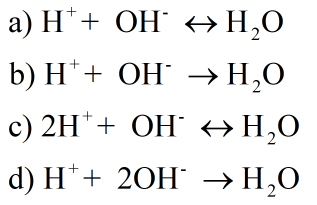Net ionic equations homework Help at TutorEye

# Best Homework Help For Net ionic equations

## Top Questions

erature and pressure? Record your answer in scientific notation using 3 significant figures.
View More

## Net ionic equations

• A chemical equation is the symbolic representation of a chemical change. It includes the reactant and product molecules separated by single or double headed arrows.

• There are multiple ways to write a chemical equation; molecular equation, ionic equation, net ionic equation.

• The molecular equation is the equation in which all the reactant and product molecules are represented as molecules. The physical state of each reactant and product molecule must be mentioned as subscript in parenthesis.

• The ionic equation is the ionic form of all the molecules of reactant and products. The molecules that exist in aqueous state must be written in their ionic form whereas the molecules in solid, liquid and gaseous state will remain in their molecular form in the ionic equation.

• The net ionic equation  is the chemical equation which shows  only those elements , compounds and ions that are directly involved in the chemical reaction and the general rule  is that if you balance  the  molecular  equation properly , the net ionic equation  will come by balancing both mass and charge.

• The spectator ions must not be part of a net ionic equation. The net ionic equation is used to balance the redox reactions mainly as it gives ideas about the oxidizing and reducing agents.

## Net Ionic Equations Questions

Question 1: Net ionic equation is done by doing _______________ mass and charge

a) Unbalancing

b) Balancing

c) Changing atoms

d) Increasing charge

Solution : The net ionic equation  will come by balancing both mass and charge.

Question 2: The net ionic equation  shows those elements involving ___________ in chemical reaction

(a) Indirectly

(b) Directly

(c) Not related to reaction

(d) Precipitated compound

Solution : The net ionic equation shows those elements involved directly in a chemical reaction.

Question 3: Which of the following equations must be the net ionic equation of a neutralization reaction of a strong acid and strong base?Solution : The net ionic equation shows those elements involved directly in a chemical reaction. So it must be b) as salt formed and strong acid-base must form ions and will be spectator ions.

### How do you write a net ionic equation?

• Write the balanced molecular equation
• Mention the state of each substance (solid, liquid, gas or aqueous
• Break the soluble ionic compounds into their ions, write charge of ion
• coefficients should be used to represent the number of ion
• Remove the spectator ions from the equation (ions which are similar on both sides).

### What is the net ionic equation for MG and HCl?

Magnesium reacts with hydrogen chloride to form magnesium chloride and hydrogen gas.The ionic equation can be written asThe net ionic equation can be written as-### How do you write a net ionic equation with water?

Molecular equation for reaction between water and sodium hydroxide can be written as-Ionic equation can be written asNet ionic equation can be written by removing spectator ions from both sides of the reaction### How is a net ionic equation written from a complete ionic equation?

To write net ionic equation from complete ionic equation one has to cancel the spectator ions on both the sides of the reaction

For example the complete ionic equation for the reaction between magnesium and hydrochloride is-The net ionic equation can be written as by removing the spectator ion which is chloride in this case.